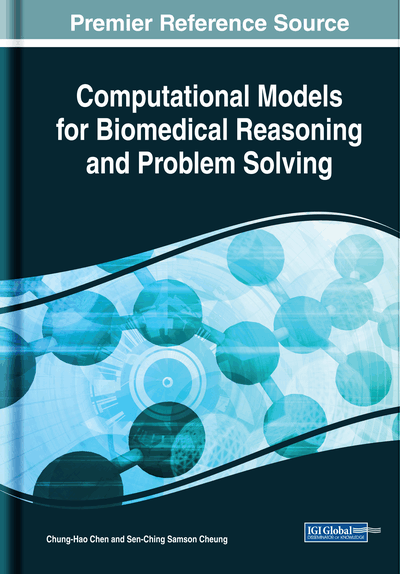# Sequential Importance Sampling for Logistic Regression Model

Ruriko Yoshida (Naval Postgraduate School, USA), Hisayuki Hara (Doshisha University, Japan) and Patrick M. Saluke (Naval Postgraduate School, USA)
DOI: 10.4018/978-1-5225-7467-5.ch009
Available
\$37.50
No Current Special Offers

## Abstract

Logistic regression is one of the most popular models to classify in data science, and in general, it is easy to use. However, in order to conduct a goodness-of-fit test, we cannot apply asymptotic methods if we have sparse datasets. In the case, we have to conduct an exact conditional inference via a sampler, such as Markov Chain Monte Carlo (MCMC) or Sequential Importance Sampling (SIS). In this chapter, the authors investigate the rejection rate of the SIS procedure on a multiple logistic regression models with categorical covariates. Using tools from algebra, they show that in general SIS can have a very high rejection rate even though we apply Linear Integer Programming (IP) to compute the support of the marginal distribution for each variable. More specifically, the semigroup generated by the columns of the design matrix for a multiple logistic regression has infinitely many “holes.” They end with application of a hybrid scheme of MCMC and SIS to NUN study data on Alzheimer disease study.
Chapter Preview
Top

## Introduction

Sampling from two-way and multiway contingency tables has a wide range of applications such as computing exact p-values of goodness-of-fit, estimating the number of contingency tables satisfying given marginal sums and more (Besag and Clifford 1989; Y. Chen et al. 2005; Diaconis and Efron 1985; Guo and Thompson 1992). For some problems, such as sparse tables, the data of interest does not permit the use of asymptotic methods. In such cases, one can apply Monte Carlo Markov Chain (MCMC) procedures using Markov bases (Diaconis and Sturmfels 1998). In order to run MCMC over the state space, all states must be connected via a Markov chain. A Markov basis is a set of moves on all contingency tables (the state space) guaranteed to be connected via a Markov chain (Diaconis and Sturmfels 1998). One important quality of a Markov basis is that the moves will work for any marginal sums under a fixed model. The two major advantages to using a MCMC approach, if a Markov basis is already known, is that it is easy to program, and it is not memory intensive. However MCMC methods are not without drawbacks where one bottleneck is the computation of a Markov basis. In fact, for 3-way contingency tables with fixed 2-margins, De Loera and Onn (2005) showed that the number of Markov basis elements can be arbitrary. To try to circumvent the difficulty of computing a Markov basis which may be large, Yuguo Chen et al. (2005; Bunea and Besag 2000) studied computing a smaller set of moves by allowing entries of the contingency table to be negative. The trade off to this approach is longer running time of the Markov chains. Even using a standard MCMC approach, to sample a table independently from the distribution, the Markov chains can take a long time to converge to a stationary distribution in order to satisfy the independent assumption. Lastly, it is not clear in general how long the chain must be run to converge.

## Complete Chapter List

Search this Book:
Reset